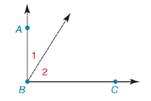Chapter 1.7, Problem 31E### Elementary Geometry for College St...

6th Edition
Daniel C. Alexander + 1 other
ISBN: 9781285195698

#### Solutions

Chapter
Section### Elementary Geometry for College St...

6th Edition
Daniel C. Alexander + 1 other
ISBN: 9781285195698
Textbook Problem
1 views

# In Exercises 27 to 35, complete the formal proof of each theorem.If the exterior sides of two adjacent acute angles form perpendicular rays, then these angles are complementary. Given: B A → ⊥ B C → Prove: ∠ 1 is comp to ∠ 2PROOF Statements Reasons 1. B A → ⊥ B C → 1. ? 2. ? 2. If two rays are ⊥ , then they meet to form a rt ∠ 3. m ∠ A B C = 90 3. ? 4. m ∠ A B C = m ∠ 1 + m ∠ 2 4. ? 5. m ∠ 1 + m ∠ 2 = 90 5. Substitution 6. ? 6. If the sum of the measures of two angles is 90 , then the angles are complementary

To determine

To find:

The complete formal proof of the given theorem.

Explanation

Given:

The statement of given theorem is,

If the exterior sides of two adjacent acute angles form perpendicular rays, then these angles are complementary.

Given: BABC

Prove: 1 is complementary to 2

The given figure is,

Definition:

If the sum of two angles is 90° then the angles are known as complementary angles.

Approach:

The formal proof of given theorem is explained in table below.

 Statements Reasons 1. BA→⊥BC→ 1. Given 2. ∠ABC is a right angle 2. If two rays are ⊥, then they meet to form a right ∠ 3. m∠ABC=90° 3. The measure of a right angle is 90°. 4. m∠ABC=m∠1+m∠2 4. Angle-addition postulate 5. m∠1+m∠2=90° 5

### Still sussing out bartleby?

Check out a sample textbook solution.

See a sample solution

#### The Solution to Your Study Problems

Bartleby provides explanations to thousands of textbook problems written by our experts, many with advanced degrees!

Get Started

#### Find the domain of the function. 5. f(x)=cosx1sinx

Single Variable Calculus: Early Transcendentals, Volume I

#### Solve the equations in Exercises 126. (x2+1)(x+1)43(x+1)73=0

Finite Mathematics and Applied Calculus (MindTap Course List)

#### In Problems 1-16, use integration by parts to evaluate the integral. 3.

Mathematical Applications for the Management, Life, and Social Sciences

#### limx1x3x21x = _____. a) 1 b) 1 c) 0 d) does not exist

Study Guide for Stewart's Single Variable Calculus: Early Transcendentals, 8th